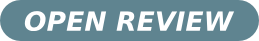# Calculation of almost all energy levels of baryons

## Authors

• Mario Everaldo de Souza Universidade Federal de Sergipe

## Keywords:

Masses of Baryons, Hadron Spectroscopy, Potential Models

## Abstract

It is considered that the effective  interaction between any two quarks of a baryon can be approximately described by a simple harmonic potential. The problem is firstly solved in Cartesian coordinates in order to find the energy levels irrespective of their angular momenta. Then, the problem is also solved in polar cylindrical coordinates in order to take into account the angular momenta of the levels. Comparing the two solutions, a correspondence is made between the angular momenta and parities for almost all experimentally determined levels. The agreement with the experimental data is quite impressive and, in general, the discrepancy between calculated and experimental values is below 5%. A couple of levels of $\Delta$, $N$, $\Sigma^{\pm}$, and $\Omega$ present discrepacies between 6.7% and 12.5% ($N(1655)$, $N(1440)$, $N(1675)$, $N(1685)$, $N(1700)$, $N(1710)$, $N(1720)$, $N(1990)$, $N(2600)$, $\Delta(1700)$, $\Delta(2000)$, $\Delta(2300)$, $\Sigma^{\pm}(1189)$, $\Lambda(1520)$, $\Omega(1672)$ and $\Omega(2250)$).

Received: 6 June 2011, Accepted: 13 July 2011; Edited by: D. Restrepo; Reviewed by: J. H. Muñoz, Universidad del Tolima, Ibagué, Colombia and Centro Brasileiro de Pesquisas Fisica; DOI: http://dx.doi.org/10.4279/PIP.030003

Cite as: M. E. de Souza, Papers in Physics 3, 030003 (2011)

## Author Biography

### Mario Everaldo de Souza, Universidade Federal de Sergipe

Department of Physics

Professor2011-08-08

## How to Cite

de Souza, M. E. (2011). Calculation of almost all energy levels of baryons. Papers in Physics, 3, 030003. https://doi.org/10.4279/pip.030003

Articles